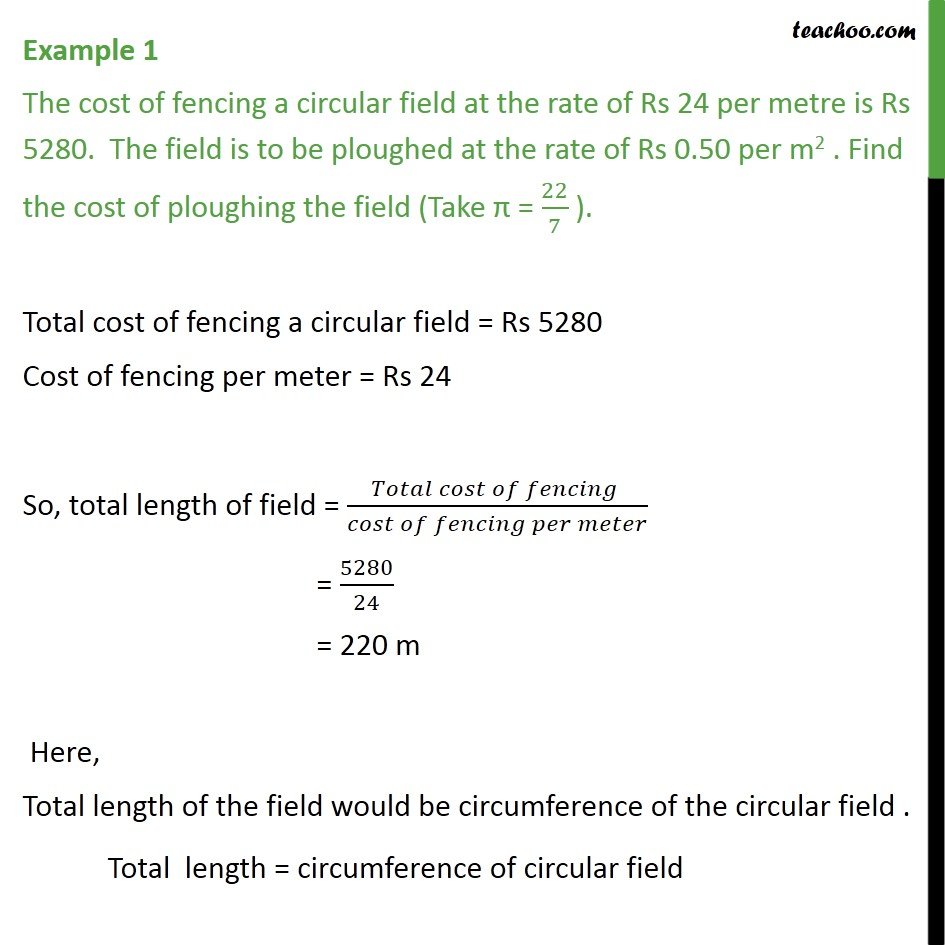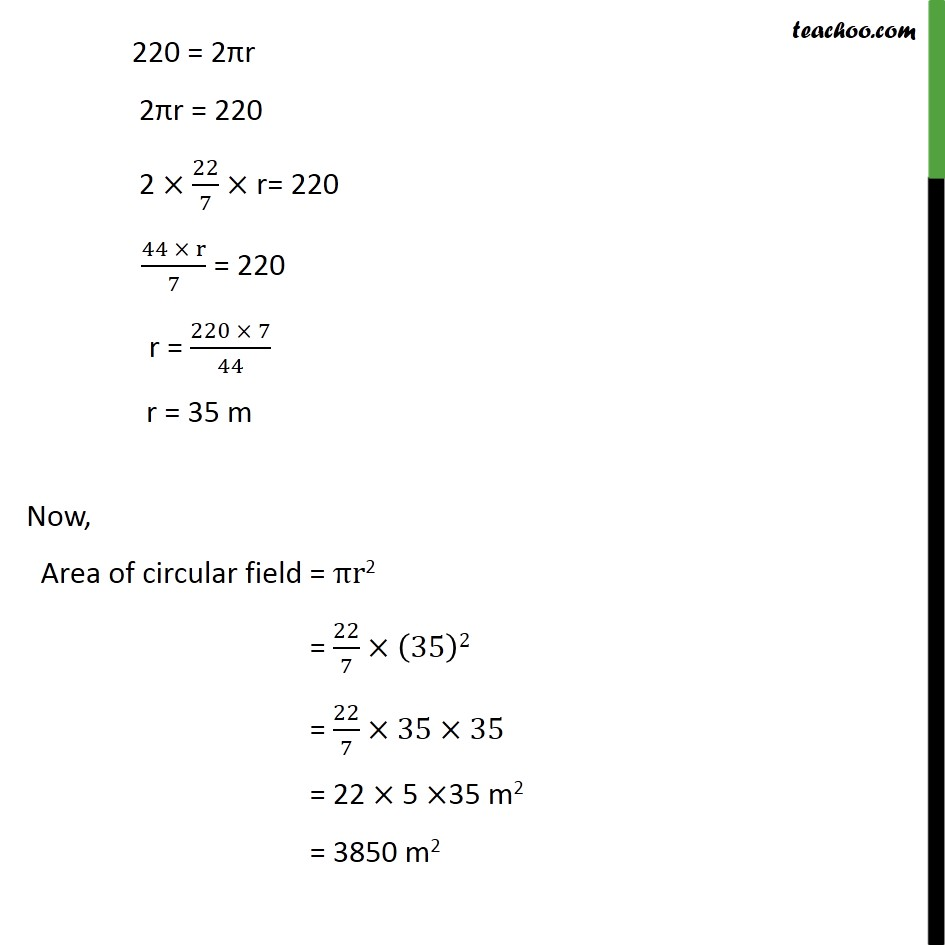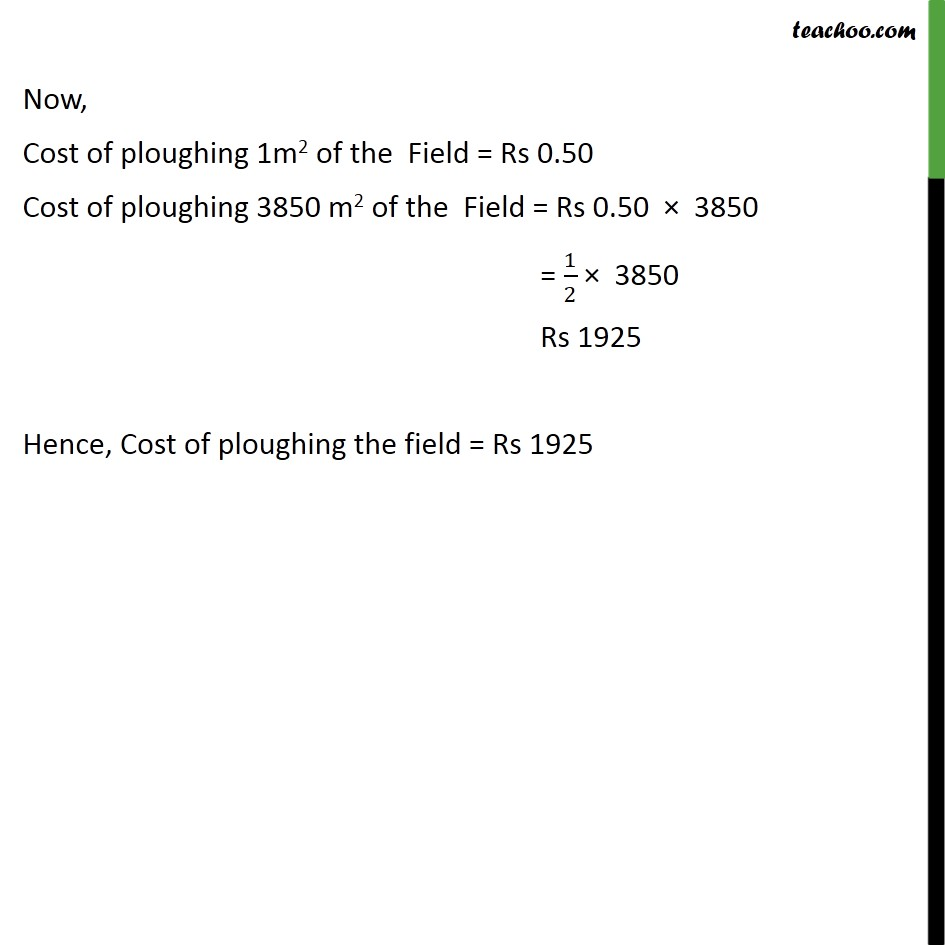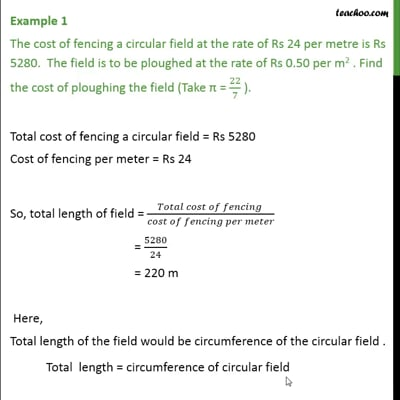Area/Perimeter of Circle

Chapter 12 Class 10 Areas related to Circles
Concept wiseThis video is only available for Teachoo black users

Introducing your new favourite teacher - Teachoo Black, at only ₹83 per month

### Transcript

Example 1 The cost of fencing a circular field at the rate of Rs 24 per metre is Rs 5280. The field is to be ploughed at the rate of Rs 0.50 per m2 . Find the cost of ploughing the field (Take π = 22/7 ). Total cost of fencing a circular field = Rs 5280 Cost of fencing per meter = Rs 24 So, total length of field = (𝑇𝑜𝑡𝑎𝑙 𝑐𝑜𝑠𝑡 𝑜𝑓 𝑓𝑒𝑛𝑐𝑖𝑛𝑔 )/(𝑐𝑜𝑠𝑡 𝑜𝑓 𝑓𝑒𝑛𝑐𝑖𝑛𝑔 𝑝𝑒𝑟 𝑚𝑒𝑡𝑒𝑟) = 5280/24 = 220 m Here, Total length of the field would be circumference of the circular field . Total length = circumference of circular field 220 = 2πr 2πr = 220 2 ×22/7× r= 220 (44 × r)/7 = 220 r = (220 × 7)/44 r = 35 m Now, Area of circular field = πr2 = 22/7×(35)2 = 22/7×35×35 = 22 × 5 ×35 m2 = 3850 m2 Now, Cost of ploughing 1m2 of the Field = Rs 0.50 Cost of ploughing 3850 m2 of the Field = Rs 0.50 × 3850 = 1/2 × 3850 Rs 1925 Hence, Cost of ploughing the field = Rs 1925